# How to use the CHOOSE Function in Excel

Excel CHOOSE function takes values and return the value corresponding to a particular index_num.
Syntax:

=CHOOSE (index_num, value1, [value2], ...)

Index_num : number corresponding to the required value
Value1 : first value
Value2 : second value

Note: the function returns a #VALUE! Error if index_num is greater than the number of values given as input.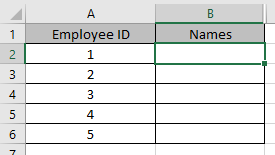Here we have some employee ID and we need their names.
Use the formula:

=CHOOSE(A2, "Anthony", "Andrew", "David", "Michelle")

A2 : index_num
Anthony : value 1
Andrew : value 2
Michelle : value 3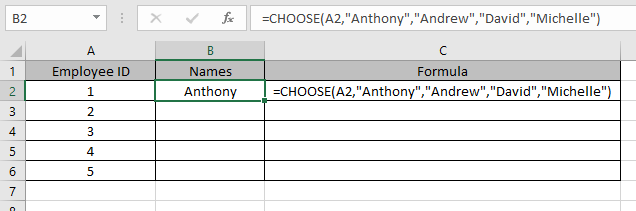As you can see from the above snapshot that values are called using index_num in argument.
Copy the formula in other cells using Ctrl + D shortcut key.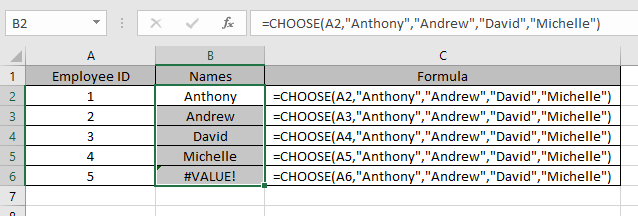This is how CHOOSE function retrieves values from the values as arguments.

CHOOSE function usually used with other functions
1. We will use the combination with Mathematical functions like SUM function
Here we have some values and needs to find the SUM of values in column B.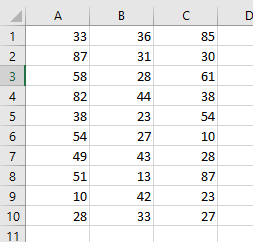Use the formula:

=SUM(CHOOSE(2, A1:A10, B1:B10, C1:C10,))

Explanation:
The CHOOSE function will calls the second value
This will drop down to
=SUM(B1:B10)
2. We will use a Combination of CHOOSE with DATE & TIME functions like MONTH function.
Use the function

=CHOOSE(MONTH(A4),"Jan","Feb","Mar","Apr","May","Jun","Jul","Aug","Sept","Oct","Nov","Dec")

Explanation:
MONTH function returns a number and the number will be the index_num for the CHOOSE function.
Values corresponding to the number will be the return value of the formula.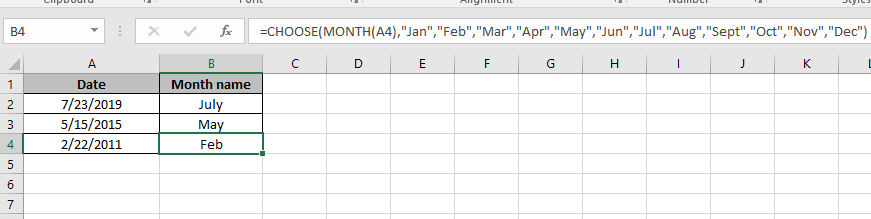As you can see here, you can rename the Month names as per your convenience.
3. We will use a Combination of CHOOSE with LOOKUP functions like VLOOKUP function.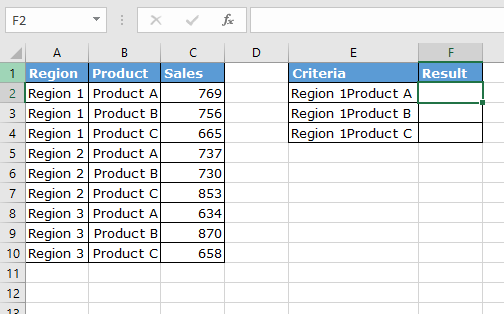Use the formula in F2 cell:

{ =VLOOKUP (E2, CHOOSE ({1,2}, A2:A10 & B2:B10, C2:C10), 2, 0) }

Note : Do not try to put curly braces manually. Use Ctrl + Shift + Enter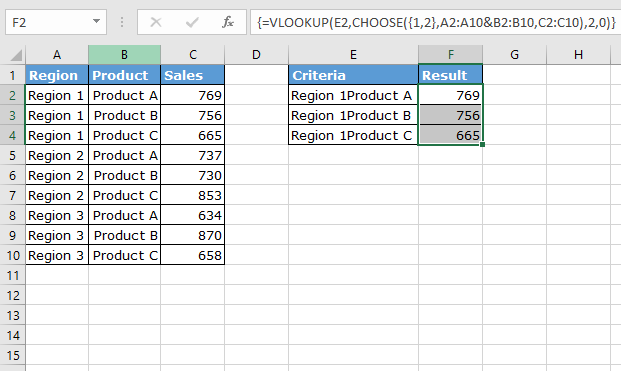As you can see CHOOSE function is very helpful when combined with other functions.
Hope you understood how to use CHOOSE function in Excel. Explore more articles on Excel Date & Time functions here. Please feel free to state your query or feedback for the above article.

Related Articles:

How to Use SWITCH function in Excel

How to Use LOOKUP function in Excel

Excel IF function

Popular Articles:

50 Excel Shortcuts to Increase Your Productivity

How to use the VLOOKUP Function in Excel

How to use the COUNTIF in Excel 2016

How to use the SUMIF Function in Excel

Terms and Conditions of use

The applications/code on this site are distributed as is and without warranties or liability. In no event shall the owner of the copyrights, or the authors of the applications/code be liable for any loss of profit, any problems or any damage resulting from the use or evaluation of the applications/code.